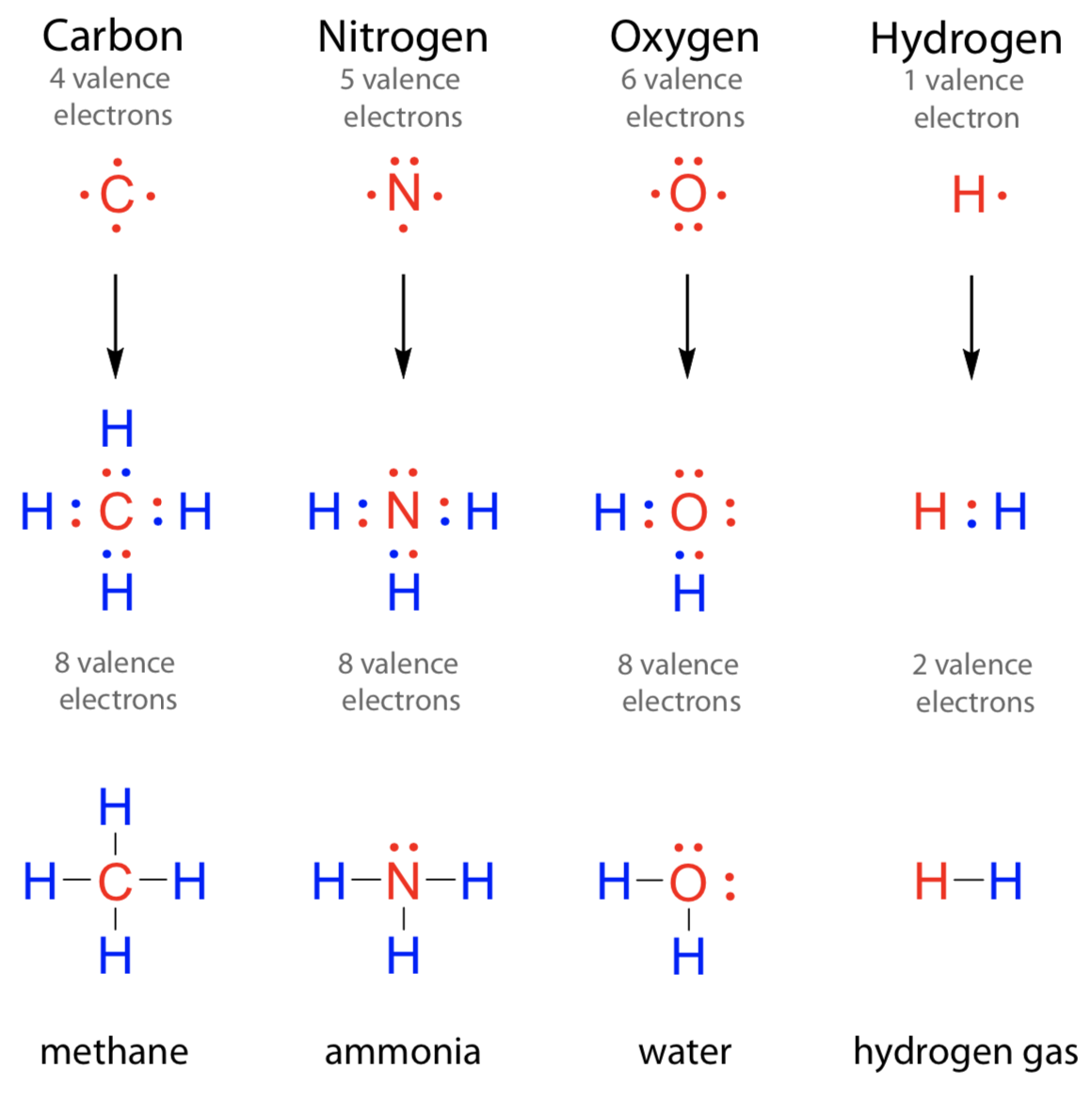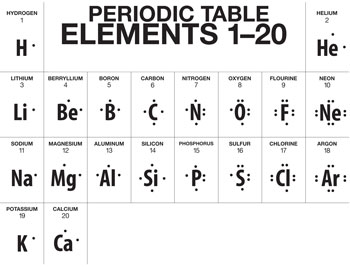# Electron Dot Diagrams Study Guide

INTRODUCTION

Oxygen forms H2O, carbon forms CH4, while boron forms BH3. The question is, how are different compounds formed? Is there an easy way to analyze how many bonds are going to be formed of an element with another one?Yes, with the help of electron dot diagrams. In this lesson, we will discuss the electron dot structure of C (Carbon) and other elements.

### WHAT ARE ELECTRON DOT DIAGRAMS?

When valence electrons are arranged around an atomic symbol, then it is called an electron dot diagram or Lewis dot structure.Valence electrons are electrons present in the outermost shell or highest occupied principal energy level and can be found out easily by the electronic configuration of an element.

For example, Li has a 1S² 2S¹ configuration. Here, the outermost shell contains 1 electron that is 2S¹. For Be, the configuration is 1S² 2S², and the number of valence electrons is 2. Valence electrons remain the same when we move from top to bottom in a periodic table.

For example, valence electrons for alkali metals that are group 1: Li, Na, K, Rb, Cs is 1, while for alkaline earth metals (group 2) such as Be, Mg, Ca, Sr, and Ba is 2. For group 13, valence electrons are 13-10 = 3, and the same pattern is followed till group 18. But valence electrons increase by 1 when we move left to the right in a periodic table.

For example, valence electrons for Li are 1, and it increases by 1 and reaches a maximum value of 8 when we move from Li to Ne in period 2.

Valence electrons are arranged because those electrons take part in chemical reactions.

Electron dot diagram for Li is: Li•
Lewis dot diagram for Be: •Be•Electrons are evenly distributed around an atom because they repel each other till carbon, electrons are arranged on 4 sides of the atomic symbol.Source

After carbon, electrons are paired up.Source

And the same pattern is followed throughout:Source

## CONCLUSION:

• When valence electrons are arranged around an atomic symbol, then it is called an electron dot diagram.
• Valence electrons are electrons present in the outermost shell.
• Electron dot diagrams are used to show bonding by depicting valence electrons are these electrons are responsible for bonding.

### FAQs:

1. What is an electron dot diagram?

When valence electrons are arranged around an atomic symbol, then it is called an electron dot diagram.

2. Write down the electronic configuration of Be and its electron dot diagram.

For Be, the electronic configuration is 1S² 2S², and the number of valence electrons is 2. So, the electron dot diagram for Be is: •Be•

3. Why are valence electrons shown in the electron dot diagram?

Because valence electrons take part in a reaction, or they are the ones that make bonds.

We hope you enjoyed studying this lesson and learned something cool about Electron Dot Diagrams! Join our Discord community to get any questions you may have answered and to engage with other students just like you! Don’t forget to download our App to experience our fun VR classrooms – we promise it makes studying much more fun! 😎.

## SOURCES:

1. Electron Dot Diagram: https://www.ck12.org/c/chemistry/electron-dot-diagrams/lesson/Electron-Dot-Diagrams-CHEM/?referrer=concept_details. Accessed 5th March 2022.
2. Lewis Electron Dot Diagram: https://www.brightstorm.com/science/chemistry/chemical-bonds/lewis-electron-dot-diagram/#:~:text=Lewis%20Electron%20Dot%20Diagrams%20are,orbital%20and%203%20p%20orbitals). Accessed 5th March 2022.
]]>